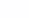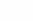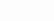# Roman numbers or how not to remember diphthongs

Open almost any implementation of converting numbers from the Arabic system to the Roman system and with almost 100% probability you will see the famous diphthongs “CM” (900), “CD” (400) and so on. And at first it seems that you can’t do without them. But that’s not true!

In fact, you can do without them and there is quite simple, beautiful mathematics behind this process. What I want to talk about today.

## A few rules and explanations

If at some point you realize that there are only 3 unique numbers in Roman numbering, then everything becomes a little simpler:

1. “I”

2. “V”

3. “X”

Everything else is obtained by combining them and multiplying byaccording to the rule off by one. For example, “CM” is just “IX”, that is, multiplication occurs by the degree in which position we expect to see this number in Arabic notation. Moreover, since the notation “IX” is equivalent to “10 – 1”, then when multiplying these brackets can be expanded: “I”“C”, “X”“M” and compose in the same sequence.

This is an extremely simple trick, but this is the difficulty – to see this pattern and understand how to implement it.

## Let’s take a look at the code

First, let’s define our number constants in both numberings

const integers = [1000, 500, 100, 50, 10, 5, 1]
const literals="mdclxvi"

const arabicToRomanMap = new Map(integers.map((int, index) => [int, literals[index]]))

And then we implement the converter

function toRoman(value: number): string {
if (!Number.isInteger(value)) throw new Error(Expected integer, got ${value}) if (value <= 0 || value >= 5000) throw new Error(Expect value between 0 and 5000 exclusive, got${value})

if (arabicToRomanMap.has(value)) return arabicToRomanMap.get(value)!

return value
.toString()
.split('')
.map(Number)
.map(processArabicDigit)
.map((digit, index, array) => processRomanDigit(digit, array.length - index))
.join('')
}

Let’s figure out what’s going on here, piece by piece.

value
.toString()
.split('')
.map(Number)

This code simply turns a number into an array of digits: 4956 becomes [4, 9, 5, 6]. And then the magic begins 🙂

Let’s look at the implementation of the function processArabicDigit :

const processArabicDigit = (digit: number) => {
if (digit === 0) return ''
if (arabicToRomanMap.has(digit)) return arabicToRomanMap.get(digit)!

const foundLessByOne = integers.find(integer => integer - digit === 1)
if (foundLessByOne !== undefined) return i\${arabicToRomanMap.get(foundLessByOne)}

return integers.reduce((accumulator, integer) => {
while (digit >= integer) {
accumulator += arabicToRomanMap.get(integer)
digit -= integer
}

return accumulator
}, '')
}

The main purpose of this function is to turn any Arabic numeral into a sequence of basic Roman numerals: “I”, “V”, “X”.

• 0 will give an empty string

• 1 and 5 basic

• 4 and 9 obey the rule off by one. They are exactly equal to “I{digit + 1}”

• 2, 3, 6, 7, 8 will go through the greedy algorithm and turn into “II”, “III”, “VI”, “VII”, “VIII” respectively.

That is, the original number 4956 will turn into [“IV”, “IX”, “V”, “VI”]. It remains to carry out the potentiation operation, which is carried out by the function processRomanDigit:

const processRomanDigit = (digit: string, position: number) => {
if (position === 4) return 'm'.repeat(toArabic(digit))

return digit
.split('')
.map(char => romanToArabicMap.get(char)!)
.map(digitNumber => arabicToRomanMap.get(digitNumber * 10 ** (position - 1))!)
.join('')
}

notice, that position = 4 is an edge case. That is, designing a number of thousands falls outside the rule, but this is not scary.

The remaining numbers are divided into their base numbers and multiplied by 10 in the desired position:

• “I” with position 4 will become “MMMM”

• “IX” with position 2 will become “CM” (discussed in example explanation)

• “V” with position 1 will become “L”

• “VI” with position 0 will remain unchanged

The last step is to put everything together. Total, 4956 will turn into “MMMMCMLVI”

## Why is this approach needed at all?

There are several reasons for this, and one of them is it’s just fun. Do not take any rules on faith and get to the bottom of the truth of things, how you can generalize the rule or derive it.

In addition, implementations through memorizing diphthongs don’t scale well if anyone ever wants to expand the domain of Roman numerals beyond 5000. But here, it’s just about making up letters, taking the following values ​​(5000, 10000) and that’s it. And so on ad infinitum.

Even that hardcoded meaning position = 4 can be generalized. And there won’t be any problems with the reverse conversion either, you just need to figure out how to generalize the regular routine for checking a valid Roman numeral. But that’s a completely different story 🙂

All the code, including the reverse conversion, can be found in repository.